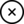Become a member of our Forum, and gain access to a lot more information!

# Lenz's Law

### Introduction

Lenz's Law is a direct result of an induced EMF being impressed on a closed Conductor. Lets take an example.1. We have a primary Coil (Coil A shown above as L1) that has a Voltage of 10 Volts Alternating Current being driven into it.
2. An Amp Meter is attached to Coil A so we can see the change in Current on Coil A.
3. A second Coil is introduced (Coil B shown above as L2) that has the same amount of turns on it. Giving a 1:1 Ratio. We will assume a perfect Circuit/Transformer with no losses.
4. We have a Variable Resistive load attached to the Secondary Coil (Coil B). Currently a switch in the circuit is open and Coil B sees no load.
5. We now close the switch, the Variable Resistance is set to an infinite value.
6. Keeping an eye on our input amp meter, and slowly winding the Variable Resistance down from infinity to 100 Ohms we can see the input Current increase slowly.
7. In an Ideal Circuit, we can see that the input Current on Coil A is proportional to the Variable Resistance that is adjusted on Coil B. This is through Ohms Law P = V^2/R

This is Lenz's law. Why is this so and How does this work?

### How and Why?

Lenz's Law in the above example is only apparent if Current is flowing in Coil B, the secondary Coil.

In Magnetics, it can be shown that, Current and the Magnetic Field follow the Right Hand Rule. Voltage, however, typically is in the opposite direction to the Current. So it can be said that Current Flow in the Conductor directly is the result of the Magnetic Field and not Voltage.

If the Coils, A and B both have Current flowing in each of them, Coil B because of the Impressed EMF from Coil A, through Faradays Law of Induction, and because we have a resistive load connected (100 Ohms), then we have a situation arise where the Current flowing will create a Magnetic Field in the Reverse Direction to the Primary Coil, Coil A, that impressed the EMF on the Secondary Coil, Coil B.

This means as Coil A sees its field move in strength from G0 to Gmax (Being the Maximum Field Strength in Gauss) this electromagnetic field will be opposed by the electromagnetic field of the Secondary Coil, Coil B at the same time, with a Magnetic Field of the Same initial Value. This Value must be overcome by the Primary Coil, Coil A and for this reason, the Input Current must increase.

So now we can see why there is such an effect as Lenz's Law. Its a pushing match, to see who is the most macho, Coil A or Coil B. One could say its a Battle of the Currents!

### How deep does Lenz's Law go?

Typically Lenz's Law only ever investigates a single Primary Coil, and a single Secondary Coil. I don't understand why this has never been bought up in Text Books or in Science Forums. Lenz's Law is a fantastic Law to study. So we know our Secondary Coil needs to have its electromagnetic field overcome by the primary electromagnetic field for this example to work as a transformer! This means the Primary Current must increase to supply the Field to the Secondary through Faradays Law of Induction.

I don't think the Human Race has investigated Lenz's Law far enough!

I can think of several ways to reduce or negate it. One thing is obvious Current must flow in the conductors or the device we are using will simply stop working!

### Can Lenz's Law be negated or overcome?

If anyone had a 100% solid sure solution here it could just be the magic pill the human race needs to evolve. We all know we need to!

Magnetic Fields have strange effects. Magnetic Fields can be manipulated by other Magnetic Fields. So here goes.

If we were to introduce not only our Primary and Secondary Coils A and B, but we also introduce another Tertiary Coil, Coil C, and use Coil C to reduce the Magnetic Field of Coil B by squeezing the field's reach down to nearly zero which is possible via superposition, then theoretically the electromagnetic Field of Coil B would have a reduced effect on Coil A. There would be a trick here, Coil C's impressed EMF would have to be sourced from Coil B's electromagnetic Field. In other words, there would need to be a Lenz's Law effect from Coil B to Coil C, that would create this superposition in the first place.

This would then reduce the Lenz's Law effect from Coil B back on Coil A.

### Related Document's:

The Jensen Unidirectional Transformer: Click Here
Magnetic Resonance by Floyd A. Sweet. PH. D: Click Here
Gennady Markov - Bi-Directional Current Transformer: Click Here
Gennady Markov - Bi-Directional Current Transformer: Patent CA2224708 Click Here
• By: hyiq.org (Chris)
• Date Created: 9/04/2014 9:44:43 AM
• Date Modified: 9/04/2014 9:57:41 AM